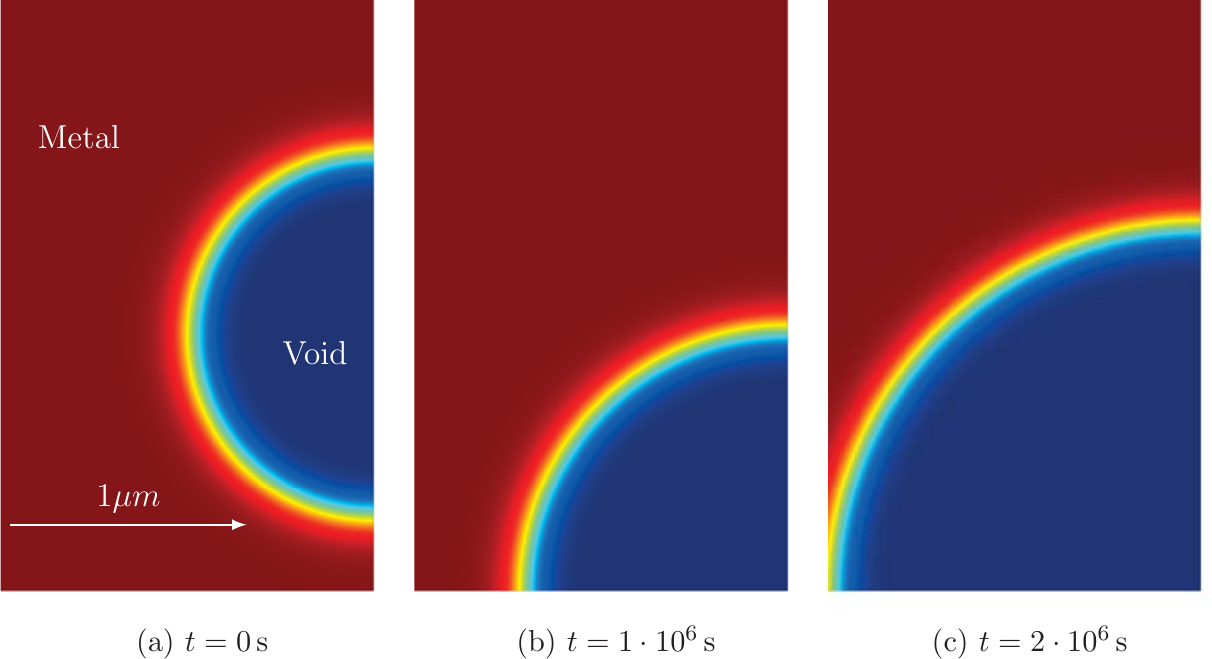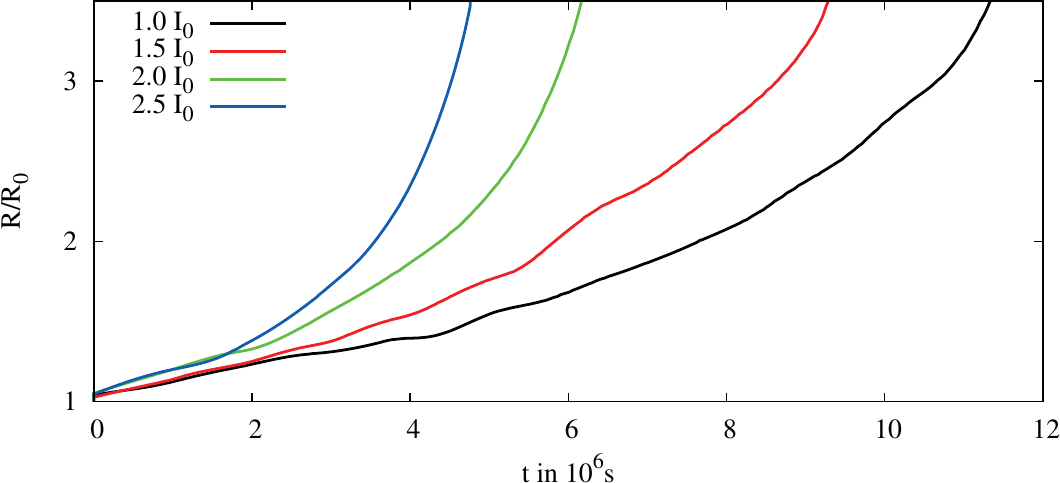Previous: 5.5 Void Growth and Evolution    Top: 5 Results and Discussion    Next: 6 Summary and Outlook

#### 5.6  Resistance Development due to Void Evolution

The simulation of the void evolution is accompanied by a resistance evaluation. In Figure 5.23 the resistance development in time is shown for different current densities. Due to the growth of the void the conducting cross section decreases leading to a higher resistance. Furthermore, the current density at the void surface increases too, leading to higher EM driving forces at the void surface accelerating the resistance growth.

Here, the failure is caused by an increase of the resistance above a certain value depending on the circuit the open TSV is used in. Therefore, a criterion has to be chosen by the circuit designers with regard to the circuit. For the fit to Black’s equation an increase of the resistance to twice the value and three times the value of the initialFigure 5.22.:  Void evolution in time. The void is placed into the structure and starts to move in the current density direction. After a certain time it has reached the end of the aluminium close to the tungsten. The circle shape deforms to a half circle to reduce the surface energy which is proportional to the surface itself. After this phase the vacancies accumulate at the void surface whereby it grows. Due to the increase of the current density along the surface the growth process gets accelerated.Figure 5.23.:  Resistance growth due to void movement and growth in the open TSV structure for different electrical currents.Figure 5.24.:  Fitting of the TTF to Black’s equation.

resistance was chosen. In Figure 5.24 the data points of the TTF for different currents for both criteria are plotted and the graphs of the two fits are sketched, again using a log-log plot. For the double value criterion a current exponent of 0.81 and for the triple value of 0.87 were obtained.

Changes in the residual stress, due to the fabrication process or surrounding elements as well as actual operating temperature distributions, have to be accounted for in order to gain meaningful TTF predictions.

Previous: 5.5 Void Growth and Evolution    Top: 5 Results and Discussion    Next: 6 Summary and Outlook